# Meeting 5 Stacks Data Structures Using C 1

• Slides: 23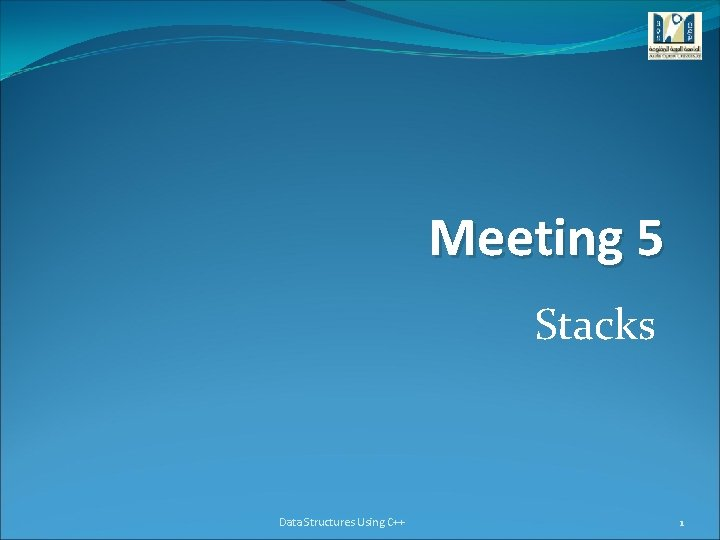Meeting 5 Stacks Data Structures Using C++ 1Meeting Five Objectives �Learn about stacks �Examine various stack operations �Learn how to implement a stack as an array �Learn how to implement a stack as a linked list �Discover stack applications �Learn to use a stack to remove recursion Data Structures Using C++ 2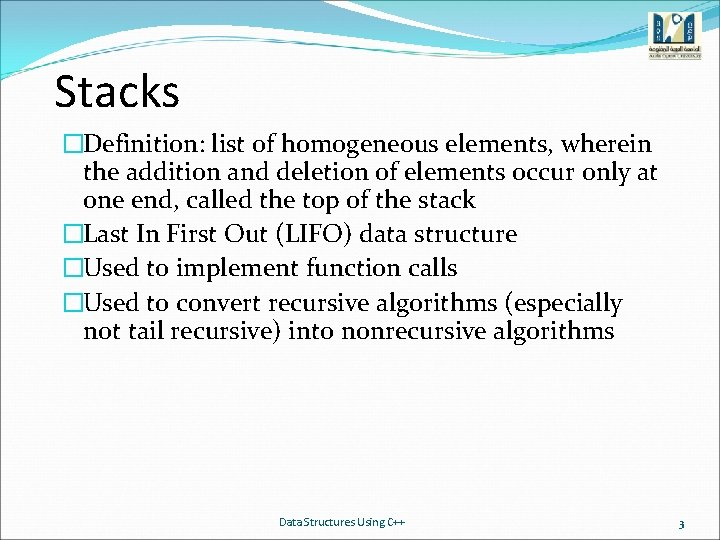Stacks �Definition: list of homogeneous elements, wherein the addition and deletion of elements occur only at one end, called the top of the stack �Last In First Out (LIFO) data structure �Used to implement function calls �Used to convert recursive algorithms (especially not tail recursive) into nonrecursive algorithms Data Structures Using C++ 3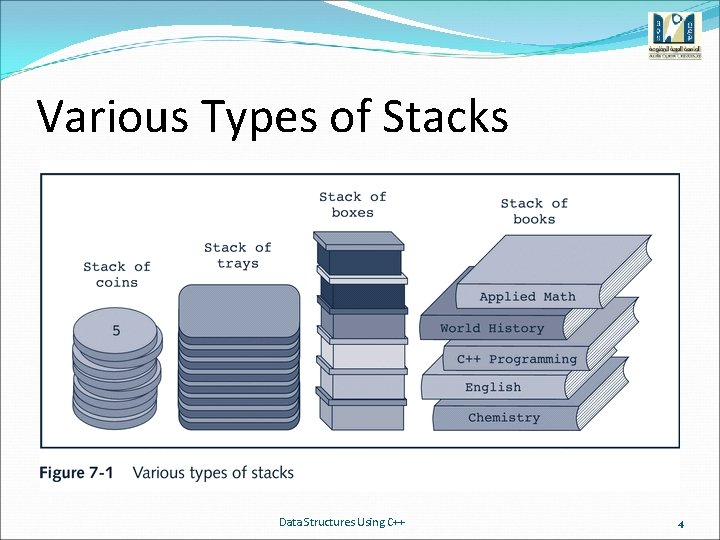Various Types of Stacks Data Structures Using C++ 4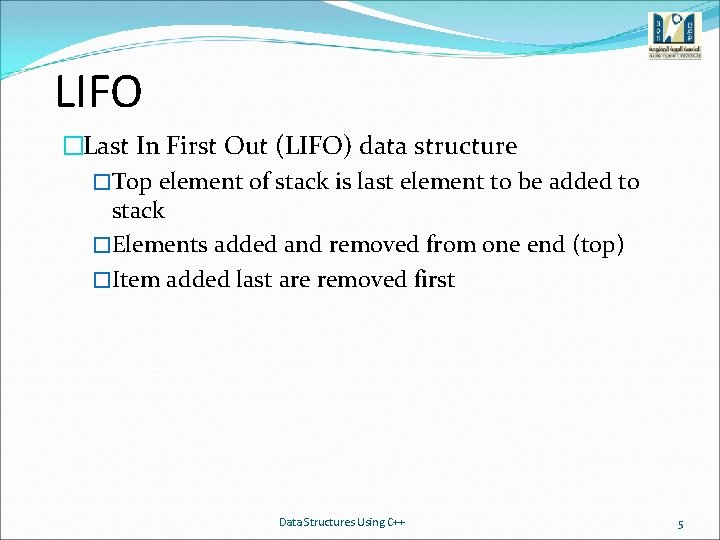LIFO �Last In First Out (LIFO) data structure �Top element of stack is last element to be added to stack �Elements added and removed from one end (top) �Item added last are removed first Data Structures Using C++ 5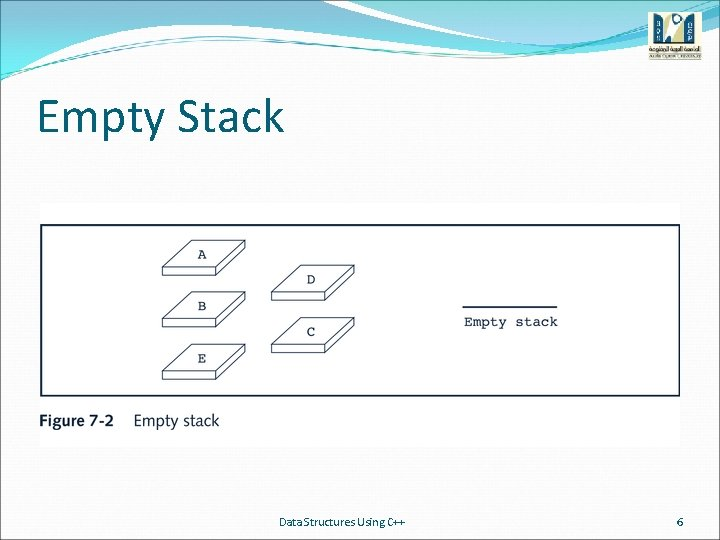Empty Stack Data Structures Using C++ 6Stack Operations Data Structures Using C++ 7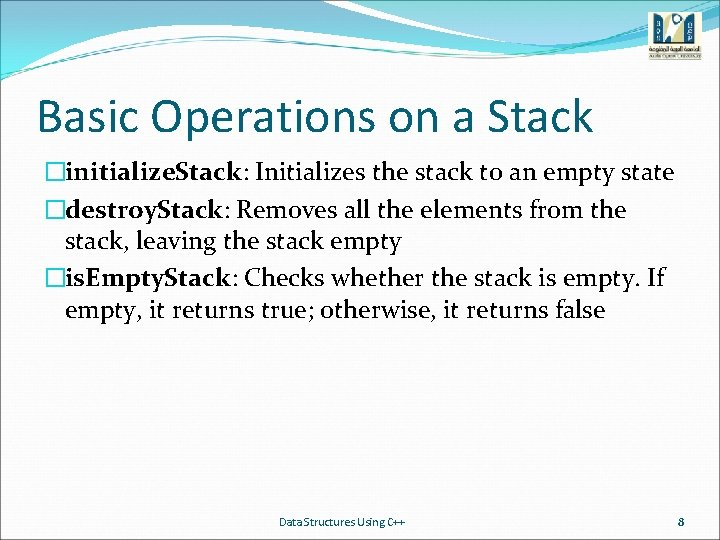Basic Operations on a Stack �initialize. Stack: Initializes the stack to an empty state �destroy. Stack: Removes all the elements from the stack, leaving the stack empty �is. Empty. Stack: Checks whether the stack is empty. If empty, it returns true; otherwise, it returns false Data Structures Using C++ 8Basic Operations on a Stack �is. Full. Stack: Checks whether the stack is full. If full, it returns true; otherwise, it returns false �push: �Add new element to the top of the stack �The input consists of the stack and the new element. �Prior to this operation, the stack must exist and must not be full Data Structures Using C++ 9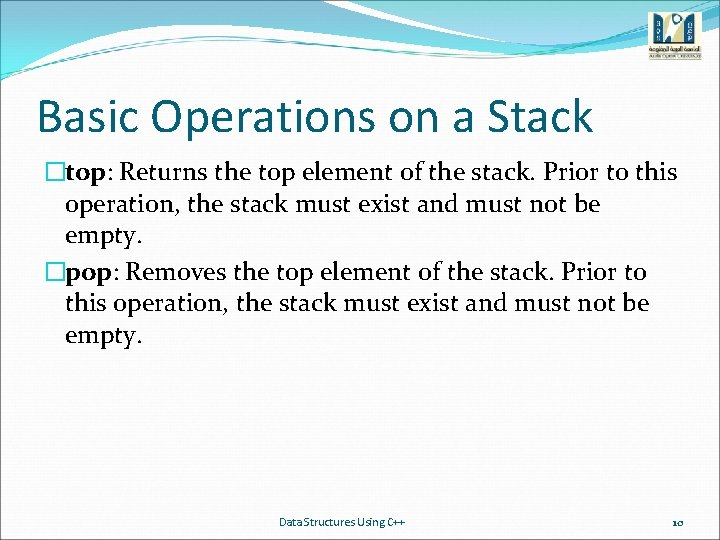Basic Operations on a Stack �top: Returns the top element of the stack. Prior to this operation, the stack must exist and must not be empty. �pop: Removes the top element of the stack. Prior to this operation, the stack must exist and must not be empty. Data Structures Using C++ 10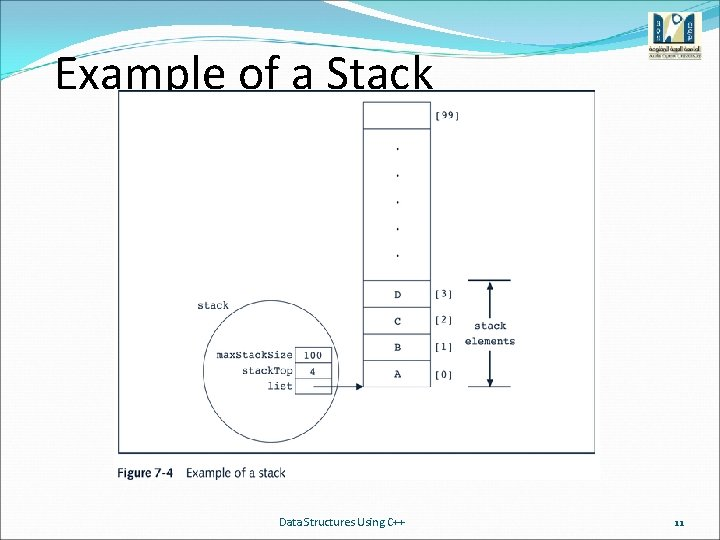Example of a Stack Data Structures Using C++ 11Empty Stack Data Structures Using C++ 12initialize. Stack and destroy. Stack template<class Type> void stack. Type<Type>: : initialize. Stack() { stack. Top = 0; }//end initialize. Stack template<class Type> void stack. Type<Type>: : destroy. Stack() { stack. Top = 0; }//end destroy. Stack Data Structures Using C++ 13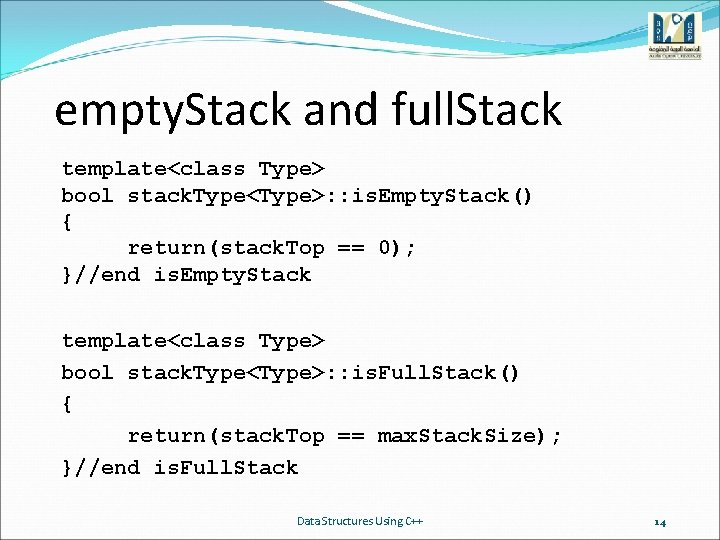empty. Stack and full. Stack template<class Type> bool stack. Type<Type>: : is. Empty. Stack() { return(stack. Top == 0); }//end is. Empty. Stack template<class Type> bool stack. Type<Type>: : is. Full. Stack() { return(stack. Top == max. Stack. Size); }//end is. Full. Stack Data Structures Using C++ 14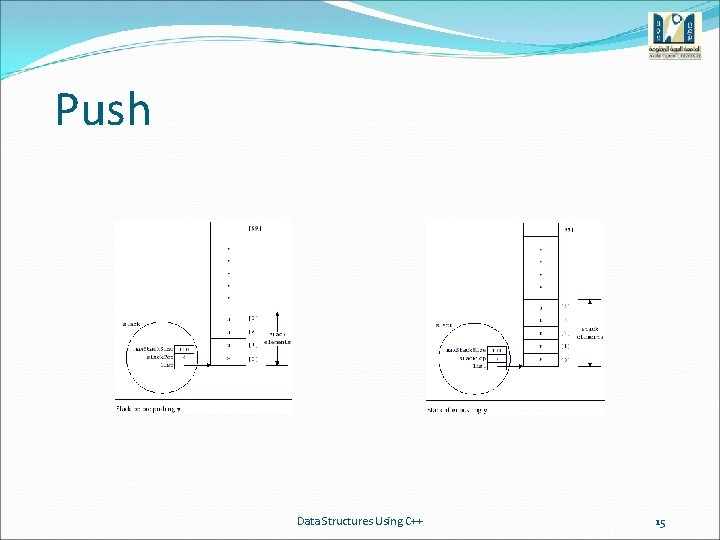Push Data Structures Using C++ 15Push template<class Type> void stack. Type<Type>: : push(const Type& new. Item) { if(!is. Full. Stack()) { list[stack. Top] = new. Item; //add new. Item at the top //of the stack. Top++; //increment stack. Top } else cerr<<"Cannot add to a full stack. "<<endl; }//end push Data Structures Using C++ 16Return Top Element template<class Type> Type stack. Type<Type>: : top() { assert(stack. Top != 0); return list[stack. Top - 1]; //if the stack is empty, //terminate the program //return the element of the //stack indicated by //stack. Top - 1 }//end top Data Structures Using C++ 17Pop template<class Type> void stack. Type<Type>: : pop() { if(!is. Empty. Stack()) stack. Top-; //decrement stack. Top else cerr<<"Cannot remove from an empty stack. "<<endl; }//end pop Data Structures Using C++ 18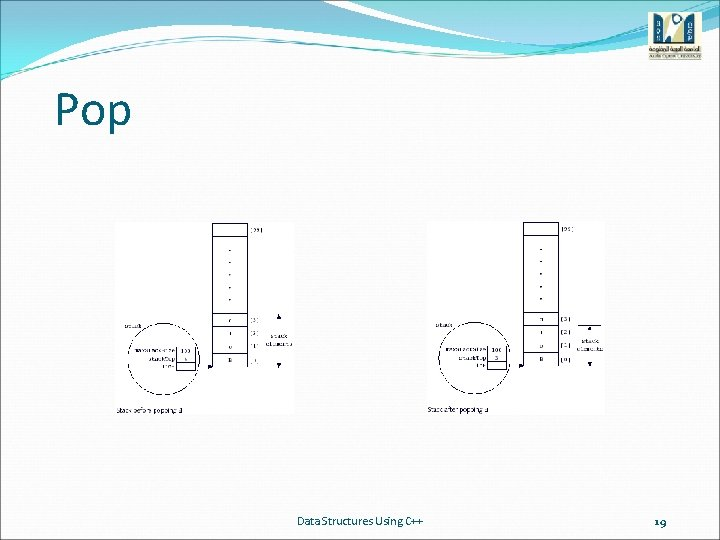Pop Data Structures Using C++ 19copy. Stack template<class Type> void stack. Type<Type>: : copy. Stack(const stack. Type<Type>& other. Stack) { delete [] list; max. Stack. Size = other. Stack. max. Stack. Size; stack. Top = other. Stack. stack. Top; list = new Type[max. Stack. Size]; assert(list != NULL); //copy other. Stack into this stack for(int j = 0; j < stack. Top; j++) list[j] = other. Stack. list[j]; }//end copy. Stack Data Structures Using C++ 20Copy Constructor template<class Type> stack. Type<Type>: : stack. Type(const stack. Type<Type>& other. Stack) { list = NULL; copy. Stack(other. Stack); }//end copy constructor Data Structures Using C++ 21Time-Complexity of Operations of class stack. Type Data Structures Using C++ 22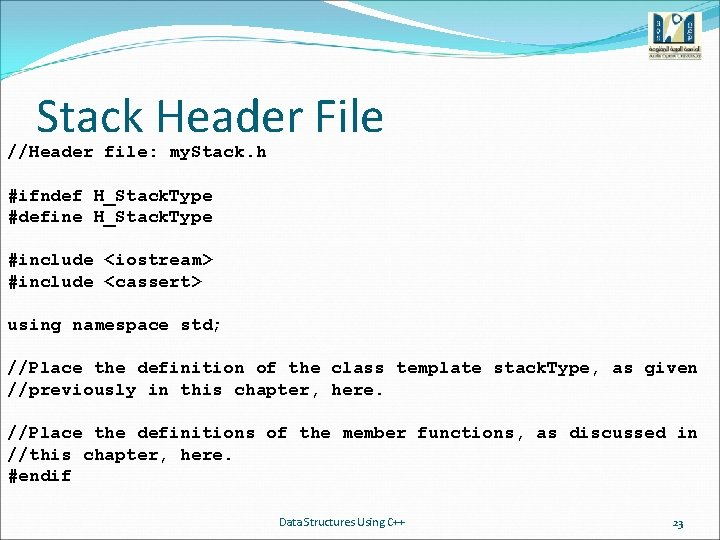Stack Header File //Header file: my. Stack. h #ifndef H_Stack. Type #define H_Stack. Type #include <iostream> #include <cassert> using namespace std; //Place the definition of the class template stack. Type, as given //previously in this chapter, here. //Place the definitions of the member functions, as discussed in //this chapter, here. #endif Data Structures Using C++ 23